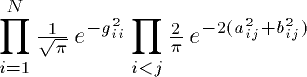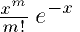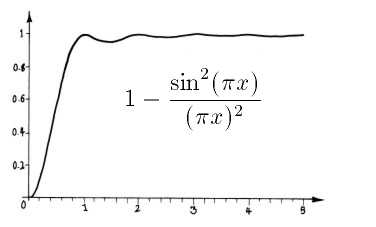The Gaussian Unitary Ensemble Hypothesis

Pólya and Hilbert conjectured (with no evidence) that the nontrivial zeros of the zeta function are of the form 1/2 + ibj, where the numbers bj are the eigenvalues of a naturally occurring Hermitian operator, hence real.

Hilbert had coined the term "spectrum" for the set of eigenvalues of a Hermitian operator by analogy with the mysterious set of lines, called spectra, which are the radiation frequencies of atoms. This was a fortuitous terminology, because later, with the development of quantum mechanics, it turned out that atomic spectra were actually spectra in the sense of operator theory - the Hermitian operators in question being the Hamiltonians of the dynamical systems representing the atoms in question.

The spectra of nuclei are more complicated than the spectra of atoms. A nucleus absorbs a neutron and somewhat later undergoes radioactive decay. The energies of such events are again quantized, but of such complexity that only a statistical understanding of their nature is attempted. This statistical line of inquiry was instituted by Wigner, pursued by Gaudin, Mehta and Dyson, and is the subject of a book, Random Matrices, by Mehta.

There are three important theories of random hermitian matrices. These model the Hamiltonians of random dynamical systems. If the dynamical system is symmetric under time reversal, then the relevant theory is that of the Gaussian Orthogonal Ensemble (GOE) or the Gaussian Symplectic Ensemble (GSE), depending on whether the total spin is an integer or half-integer. On the other hand, the Gaussian Unitary Ensemble (GUE) models random Hamiltonians without time reversal symmetry. Since this is the statistical theory which is relevant to the Riemann zeta function, this is the one we will describe.

The Gaussian Unitary Ensemble of degree N consists of the set of all NxN Hermitian matrices, together with a certain probability measure. This measure is the unique probability measure on the set of NxN Hermitian matrices which is invariant under conjugation by unitary matrices, such that the individual matrix entries are independent random variables. Note that the first hypothesis is significant, but the second is artificial. There is nothing sacred about these ensembles. Dyson has shown that the essential features of the analysis can be carried out in a more natural and general setting.

We now describe the GUE in specific terms. If i=j, the ij-th entry of the matrix g is real, while if i<j, it is complex. If i<j, let

gij = aij + ibij

The measure in question is Lebesgue measure times.

The eigenvalues of random Hermitian matrices are not randomly distributed in relation to each other. We recall the basic facts about the Poisson distribution. Suppose that a series of points are distributed at random along the line, with average density 1, and that the coordinates of the points are independent random variables. Then the probability of finding m points in a given interval of length x is.

On the other hand, the eigenvalues of a random Hermitian matrix do not behave like independent random variables, so the probability of finding more than one eigenvalue in a short interval is much less than the Poisson distribution would suggest. In short, the eigenvalues repel. This is shown by the pair correlation function. Suppose that we examine an interval on which the relative spacing of the eigenvalues has been adjusted so that the density of zeros on this interval is one. If t is an eigenvalue in the interval, the pair correlation function gives the probability density* of finding a second eigenvalue near t+x. This function is:Around 1970, Montgomery investigated the relative spacing of zeros of the zeta function. He gave evidence that the pair correlation for the zeros of the zeta function is exactly this function. He showed this to Dyson, who recognized this as the pair correlation function for random Hermitian matrices in the Gaussian Unitary Ensemble. Odlyzko gave impressive numerical evidence for the GUE hypothesis, and in a tour de force Rudnick and Sarnak showed that the "explicit formulas" that the zeros obey imply the GUE statistics; assuming the Ramanujan conjecture, they prove this distribution for zeros of Langlands L-functions. Thus on a small scale, the zeros of the zeta function are distributed like the zeros of a random Hermitian operator. On the large scale, there will be deviations from the GUE distribution related to particular prime numbers.

Gutzwiller gave a trace formula in the setting of quantum chaos which relates the classical and quantum mechanical pictures. Given a chaotic (classical) dynamical system, there will exist a dense set of periodic orbits, and one side of the trace formula will be a sum over the lengths of these orbits. On the other side will be a sum over the eigenvalues of the Hamiltonian in the quantum-mechanical analog of the given classical dynamical system.

This setup resembles the explicit formulas of prime number theory. In this analogy, the lengths of the prime periodic orbits play the role of the rational primes, while the eigenvalues of the Hamiltonian play the role of the zeros of the zeta function. Based on this analogy and pearls mined from Odlyzko's numerical evidence, Sir Michael Berry proposes that there exists a classical dynamical system, asymmetrical with respect to time reversal, the lengths of whose periodic orbits correspond to the rational primes, and whose quantum-mechanical analog has a Hamiltonian with zeros equal to the imaginary parts of the nontrivial zeros of the zeta function. The search for such a dynamical system is one approach to proving the Riemann hypothesis.

* A discussion of this (perhaps confusing) point between Mike Hardy and Dan Bump can be found here.

[this is a slight adaptation of D. Bump's page at http://match.Stanford.EDU/rh/gue.html]
back to random matrices page
back to QM page